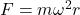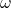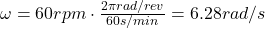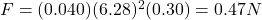## A 40 g ball rolls around a 30 cm -diameter L-shaped track, shown in the figure, (Figure 1)at 60 rpm . What is the magnitude of the net force

Question

A 40 g ball rolls around a 30 cm -diameter L-shaped track, shown in the figure, (Figure 1)at 60 rpm . What is the magnitude of the net force that the track exerts on the ball? Rolling friction can be neglected.
Hint: The track exerts more than one force on the ball.

in progress 0
6 months 2021-07-30T15:25:45+00:00 1 Answers 191 views 0

## Answers ( )

0.47 N

Explanation:

Here we have a ball in motion along a circular track.

For an object in circular motion, there is a force that “pulls” the object towards the centre of the circle, and this force is responsible for keeping the object in circular motion.

This force is called centripetal force, and its magnitude is given by:where

m is the mass of the objectis the angular velocity

r is the radius of the circle

For the ball in this problem we have:

m = 40 g = 0.04 kg is the mass of the ballis the angular velocity

r = 30 cm = 0.30 m is the radius of the circle

Substituting, we find the force: